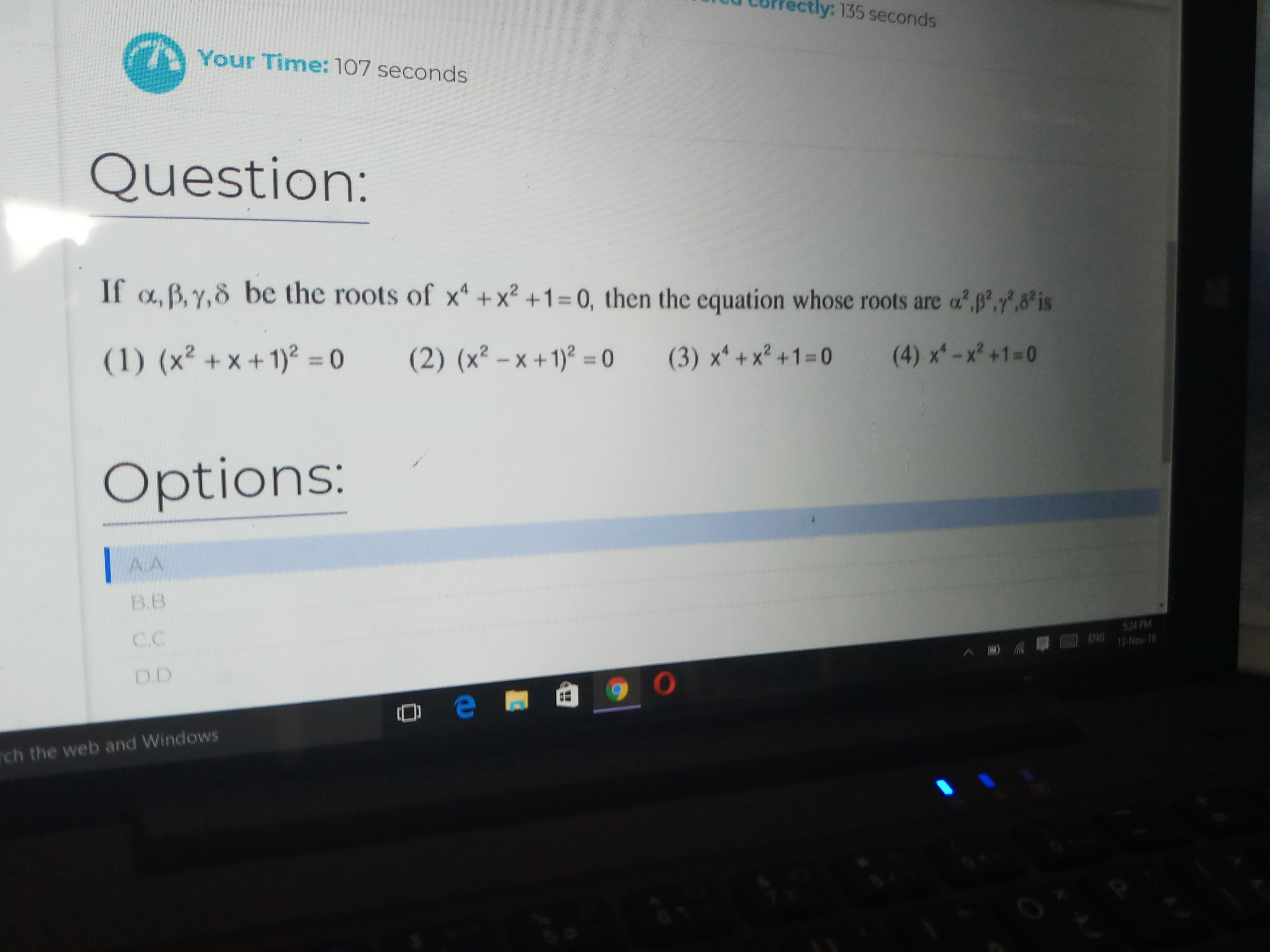# Doubt from miscellaneous equationsthe right answer is A can anyone plz explain how....

The equation whose roots are exactly equal to the square of the roots of a given equation.Then y=x²
x=√y
Substituting in the given equation, we get
(√y)⁴+(√y)²+1=0
y²+y+1=0
by squaring, (y²+y+1)²=0
Which is option (1)

2 Likes

\alpha^4+\alpha^2+1 =0 \Rightarrow \alpha^4+1 = -\alpha^2, squaring and rearranging we get

\alpha^8+\alpha^4+1=0. Hence \alpha^4 is a root of x^2+x+1=0 and similarly the others. This should be the answer and it fits as it turns out that \alpha^4=\gamma^4 and \beta^4=\delta^4

Reason being x^4+x^2+1=(x^2-x+1)(x^2+x+1)=0 so the roots are \pm \omega, \pm \omega^2 where these are cube roots of unity.

1 Like